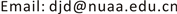﻿ 基于影子价格的排污权定价机制实证研究 Empirical Study on the Pricing Mechanism of Emission Rights Based on Shadow Price

Sustainable Development
Vol.07 No.04(2017), Article ID:22283,7 pages
10.12677/SD.2017.74020

Empirical Study on the Pricing Mechanism of Emission Rights Based on Shadow Price

Shanshan Ding, Jindong Duan

College of Economics and Management, Nanjing University of Aeronautics and Astronautics, Nanjing JiangsuReceived: Sep. 25th, 2017; accepted: Oct. 9th, 2017; published: Oct. 12th, 2017ABSTRACT

Due to the increasingly serious environmental pollution and global environmental governance and protection have become hot topic, as emissions trading to achieve an important means to promote the dual goals of economic development and protection of the ecological environment, in the main developed country environment management is playing a more and more important role. How to reasonable pricing has been the blowdown right trade key factor. This paper analyzes the advantages and disadvantages of the initial allocation of emission rights way, through the establishment of shadow price model and simulation of initial emission permits and secondary pricing mechanism, obtained initial allocation of emission rights price and sewage in secondary trading market equilibrium price can provide a reference role for the government price sell emission rights and determine the secondary market emissions trading price.1. 引言

2. 针对排污权初始分配方式的分析

2.1. 排污权初始分配方式

2.2. 初始分配方式的利弊

2.3. 我国排污权交易初次尝试限额交易

3. 标价出售的排污权定价模型的构建

3.1. 排污权初次定价模型

3.1.1. 基本假设

3.1.2. 排污权初始定价模型的分析

$\text{Max}\text{\hspace{0.17em}}{C}_{总}=\underset{i=1}{\overset{n}{\sum }}\left(C\ast {B}_{i}\right)=\underset{i=1}{\overset{n}{\sum }}\left(C\ast x\ast {b}_{i}\right)$ (1)

$\text{s}\text{.t}\text{.}\text{\hspace{0.17em}}\text{\hspace{0.17em}}\underset{i=1}{\overset{n}{\sum }}{b}_{i}\le {A}_{总}$

$D=\underset{i=1}{\overset{n}{\sum }}\left(C\ast x\ast {b}_{i}\right)+\lambda ×\left({A}_{总}-\underset{i=1}{\overset{n}{\sum }}{b}_{i}\right)$ (2)

$\text{s}\text{.t}\text{.}\text{\hspace{0.17em}}\text{\hspace{0.17em}}\underset{i=1}{\overset{n}{\sum }}{b}_{i}\le {A}_{总}$

3.2. 排污权二次交易定价模型

3.2.1. 基本假设

3.2.2. 排污权二次定价模型分析

$\text{Min}\text{\hspace{0.17em}}I=p×\left({b}_{i}-{b}_{i}^{0}-{a}_{i}\right)+C×x×{a}_{i}+{f}_{i}\left({b}_{i}^{0}\right)+{H}_{i}\left({g}_{i}\right)+{I}_{i}\left({B}_{i}\right)$ (3)

$\begin{array}{l}\partial {f}_{i}\left({b}_{i}^{0}\right)/\partial {b}_{i}^{0}+\partial {H}_{i}\left({g}_{i}\right)/\partial {g}_{i}\ast \partial {g}_{i}/\partial {b}_{i}^{0}+x\ast \partial {I}_{i}\left({B}_{i}\right)/\partial {b}_{i}\ast \partial {b}_{i}/\partial {b}_{i}^{0}+\left(C\ast x\right)\ast \partial {a}_{i}/\partial {b}_{i}^{0}-p\ge 0\\ {\gamma }^{\ast }\ast \left[\partial {f}_{i}\left({b}_{i}^{0}\right)/\partial {b}_{i}^{0}+\partial {H}_{i}\left({g}_{i}\right)/\partial {g}_{i}\ast \partial {g}_{i}/\partial {b}_{i}^{0}+\partial {I}_{i}\left({B}_{i}\right)/\partial {B}_{i}\ast \partial {B}_{i}/\partial {b}_{i}^{0}+\left(C\ast x\right)\ast \partial {a}_{i}/\partial {b}_{i}^{0}-p\right]=0\\ {b}_{i}^{0}\ge 0\end{array}$ (4)

$\partial {f}_{i}\left({b}_{i}^{0}\right)/\partial {b}_{i}^{0}+x\ast \partial {I}_{i}\left({B}_{i}\right)/\partial {B}_{i}-C\ast x=p+\partial {H}_{i}\left({g}_{i}\right)/\partial {g}_{i}$ (5)

$\partial {f}_{i}\left({b}_{i}^{0}\right)/\partial {b}_{i}^{0}+x\ast \partial {I}_{i}\left({B}_{i}\right)/\partial {B}_{i}-C\ast x=p-\partial {H}_{i}\left({g}_{i}\right)/\partial {g}_{i}$ (6)

$\partial {f}_{1}\left({b}_{1}^{0}\right)/\partial {b}_{1}^{0}+x\ast \partial {I}_{1}\left({B}_{1}\right)/\partial {B}_{1}-C\ast x=p+\partial {H}_{1}\left({g}_{1}\right)/\partial {g}_{1}$ (7)

$\partial {f}_{2}\left({b}_{2}^{0}\right)/\partial {b}_{2}^{0}+x\ast \partial {I}_{2}\left({B}_{2}\right)/\partial {B}_{2}-C\ast x=p$ (8)

$P=1/2\left[\partial {f}_{1}\left({b}_{1}^{0}\right)/\partial {b}_{1}^{0}+\partial {f}_{2}\left({b}_{2}^{0}\right)/\partial {b}_{2}^{0}+x\ast \left[\partial {I}_{1}\left({B}_{1}\right)/\partial {B}_{1}+\partial {I}_{2}\left({B}_{2}\right)/\partial {B}_{2}\right]\right]$ (9)

4. 要素优化配置对排污权定价模型重要性

4.1. 合法的排污权是影子价格发挥作用的前提

4.2. 环境政策的有效性制约着影子价格的检测效应

4.3. 排污权市场处于何种状态决定价格是否真实

4.4. 企业参与度反映了排污权定价的合理性

4.5. 环境信息系统影响排污权定价的精确性

5. 结束语

Empirical Study on the Pricing Mechanism of Emission Rights Based on Shadow Price[J]. 可持续发展, 2017, 07(04): 157-163. http://dx.doi.org/10.12677/SD.2017.74020

1. 1. 赵雯砚. 初始排污权定价模型研究[D]: [硕士学位论文]. 吉林大学, 2013.

2. 2. 刘钢, 王慧敏, 仇蕾, 刘高峰. 湖域工业初始排污权纳什议价模型研究——以江苏省太湖流域纺织行业为例[J]. 中国人口. 资源与环境, 2012(10): 78-85.

3. 3. 王兆群, 张华伦. 有偿分配下排污权基准价定价模型研究[J]. 环境污染与防治, 2015(1): 93-99.

4. 4. 张廷熙, 仇蕾. 排污权交易市场下的厂商最优决策[J]. 水利经济, 2015(3): 37-40 + 76-77.

5. 5. 张坤, 孙涛, 戴红军. 初始排污权定价的分散决策模型[J]. 技术经济, 2013(7): 53-56.

6. 6. 张茜, 于鲁冀, 王燕鹏, 张培. 水污染物初始排污权定价策略研究——以河南省为例[J]. 南水北调与水利科技, 2016(1): 165-171.

7. 7. 汪珊珊, 李念, 王晶晶, 李晶, 陈金毅. Black-Scholes模型在排污权初始分配定价中的应用[J]. 上海环境科学, 2013(4): 149-152.

8. 8. 罗智霞. 碳排放权交易定价研究综述[J]. 技术经济与管理研究, 2014(10): 77-81.

9. 9. 涂正革, 谌仁俊. 排污权交易机制在中国能否实现波特效应? [J]. 经济研究, 2015(7): 160-173.

10. 10. 肖加元, 潘安. 基于水排污权交易的流域生态补偿研究[J]. 中国人口∙资源与环境, 2016(7): 18-26..

11. 11. 金帅, 盛昭瀚, 杜建国. 转型背景下排污权初始分配机制优化设计[J]. 中国人口.资源与环境, 2013(12): 48-56.

12. 12. 易永锡, 李寿德, 邓荣荣. 排污权价格不确定性对厂商污染治理投资决策影响的实物期权分析[J]. 系统管理学报, 2017(1): 78-84.

13. 13. 孙婷, 冯庆革, 于嵘. 广西环境监管体系存在问题与对策[J]. 大众科技, 2016(12): 35-39.

14. 14. 于杰, 周伟铎, 蒋金星. 排污权交易: 理论引进与本土化实践[J]. 中国地质大学学报(社会科学版), 2014(6): 96-104.

15. 15. 魏圣香, 王慧. 美国排污权交易机制的得失及其镜鉴[J]. 中国地质大学学报(社会科学版), 2013(6): 34-39.

16. NOTES



1库兹涅茨曲线是上世纪50年代诺贝尔奖获得者、经济学家库兹涅茨用来分析人均收入水平与分配公平程度之间关系的一种学说。研究表明，收入不均现象随着经济增长先升后降，呈现倒U型曲线关系。当一个国家经济发展水平较低的时候，环境污染的程度较轻，但是随着人均收入的增加，环境污染由低趋高，环境恶化程度随经济的增长而加剧；当经济发展达到一定水平后，也就是说，到达某个临界点或称“拐点”以后，随着人均收入的进一步增加，环境污染又由高趋低，其环境污染的程度逐渐减缓，环境质量逐渐得到改善，这种现象被称为环境库兹涅茨曲线。